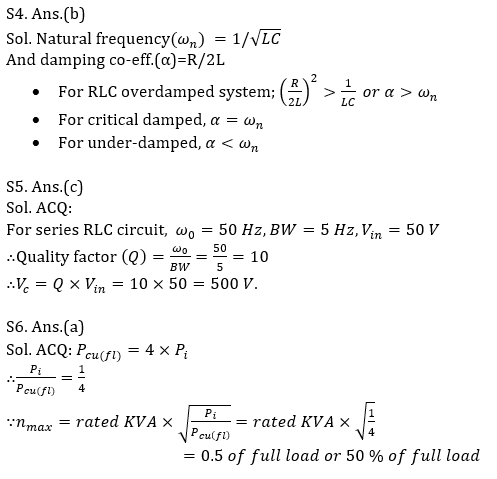Engineering Jobs   »   QUIZ DFCCIL ELECTRICAL QUIZ

# DFCCIL’21 EE: Daily Practices Quiz 27-July-2021

Each question carries 1 mark.
Negative marking: 1/4 mark
Total Questions: 06
Time: 08 min.

Q1. For equilateral spacing of conductors of an un-transposed 3-phase line, we have
(a)Balanced receiving end voltage and no communication interference
(b)Unbalanced receiving end voltage and no communication interference
(c)Balanced receiving end voltage and communication interference
(d)Unbalanced receiving end voltage and communication interference

Q2. In …… instruments the deflecting torque depends on the frequency
(a) Induction type instruments
(b) Hot wire instruments
(c) Moving iron instruments
(d) Moving coil instruments

Q3. Commutation conditions at full load for large DC machines can be efficiently checked by the
(a) Brake test
(b) Swinburne’s test
(c) Hopkinson’s test
(d) Field test

Q4. A series or parallel RLC circuit is said to be over damped if:
(a)α=ω_n
(b)α>ω_n
(c)α<ω_n
(d)2α=ω_n

Q5. Consider a series RLC circuit excited by a sinusoidal source of 50 V resonates at a frequency of 50 Hz. If the bandwidth is 5 Hz, what will be the voltage across the capacitor?
(a) 125 V
(b) 250 V
(c) 500 V
(d) 1000 V

Q6. The full-load copper loss of a transformer is four times its core loss. The efficiency will be maximum at _______.

SOLUTIONS

S1. Ans.(a)
Sol. If conductors are equally spaced then per phase inductance will be same and receiving end voltage will be balanced and communication interference will not take place. So here no need of transposition.

S2. Ans.(a)
Sol. for induction type instruments –
T_d=kωϕ_1m ϕ_2m sin α
ϕ_1m,ϕ_2m = maximum fluxes produced by the currents
α = phase difference between the two fluxes
So, in induction type instrument deflecting torque depends on the frequency.

S3. Ans.(c)
Sol. Hopkinson’s test is to check the commutation condition at Full-load for large DC machines.
Hopkinson’s: – Also known as Regenerative, back-to-back test and heat run testSharing is caring!